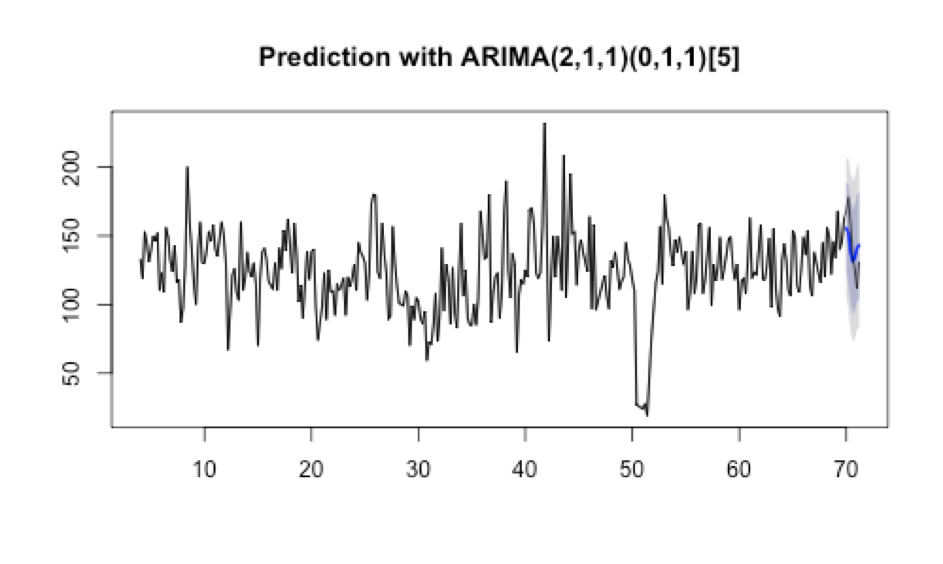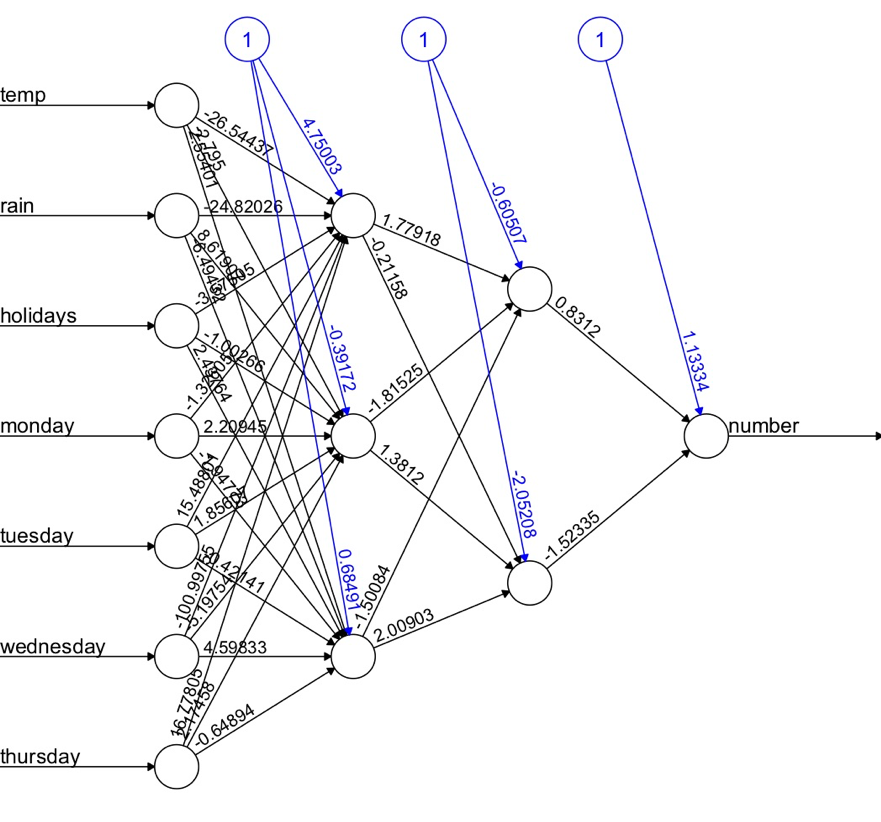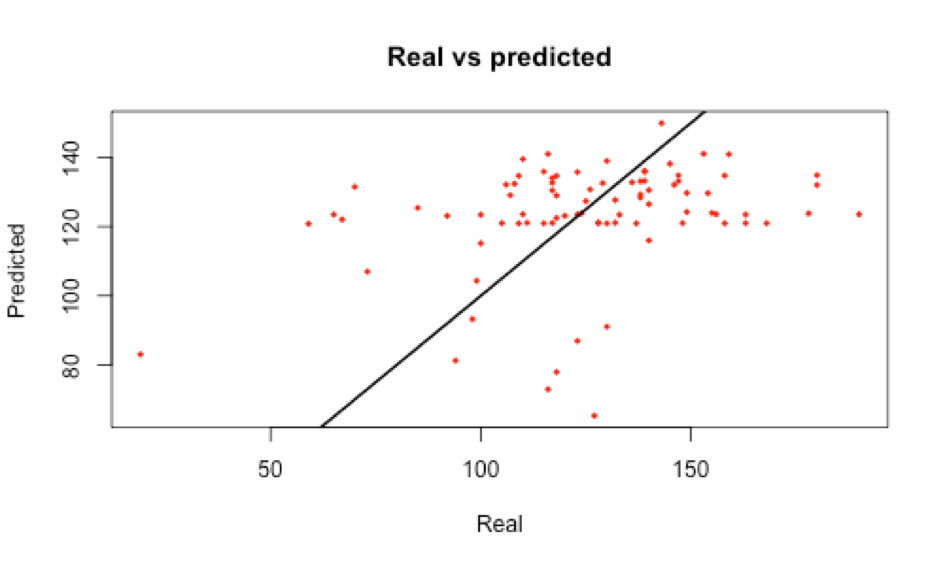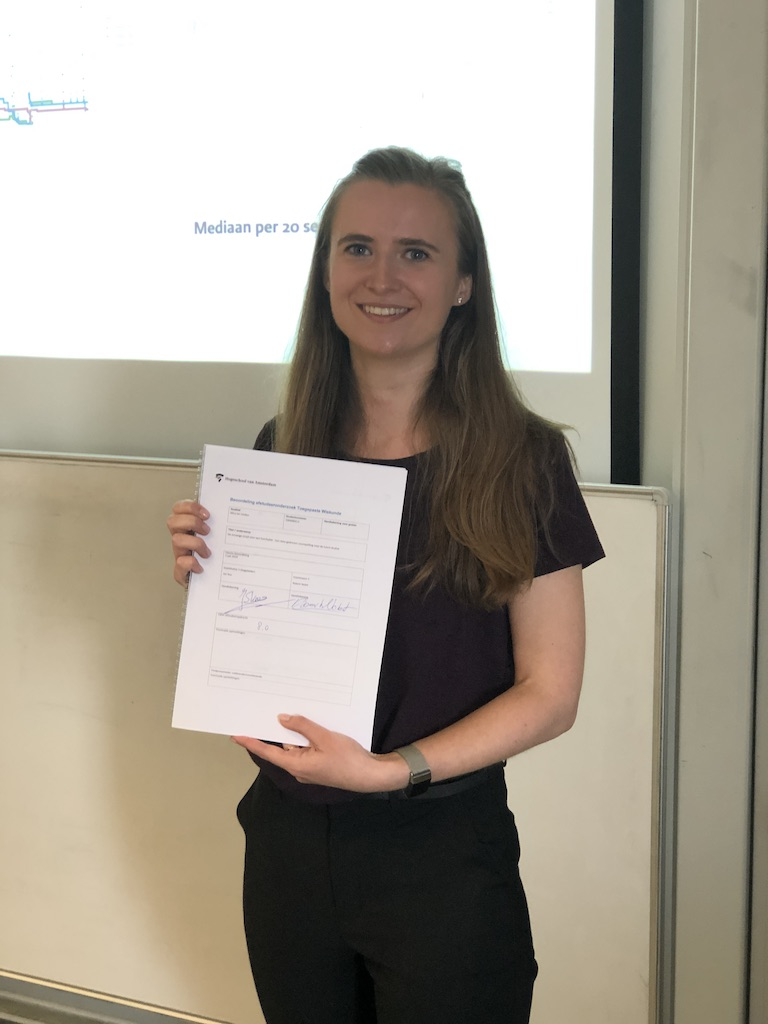Using data science to predict lunch traffic - Part 2

Auteur
Mira ter Linden
Datum

In my previous blogpost you can read how I prepared and analysed our lunch data, made plots to visualize the data and came to some interesting insights. After analysing all the data collected by our smartscales and sensors, I would like to share more results from my research in this follow-up blogpost.

The data I have analyzed is collected by our smart scales where the missing values are filled in with the data collected by the counted plates by the dishwashers. If case you want to read more about our smart scales, please read this blogpost.

A predicting model

My goal was to make a prediction model that could predict how many people are going to lunch in our office for the coming days. First I made a prediction using an ARIMA model. In the plot below the prediction represents the blue line and the black line contains the real data. The grey areas show the 80% and 95% confidence intervals. As you might notice, the black line falls in the 80% confidence interval. Besides the plot, a MAPE (Mean Absolute Percentage Error) was calculated. This is a percentage to see how accurate the prediction is. The found percentage is 11,1%; this means that the model is 89,9% accurate. Also I calculated that the average difference between the predicted values and the real data is 16 plates.Correlations?

Besides a prediction for the next days, a question I wanted to answer during my project was if there were any correlations between the number of people lunching in the office and external factors. First I had to look for factors that could be correlating with the number of people lunching per day. The following factors have been researched:

• Day of the week
• Average temperature of the day
• Average rainfall of the day
• School holidays

I used a linear regression method for this. The best model that came out can be described with the following formula:

Number of plates = 135.6 - 0.06 * temperature - 19.8 * holidays + ε

In this formula temperature is the average temperature in 0.1 degrees Celsius of that day, the holidays is 1 for a holiday day and 0 for a regular day and ε is the error term. The R-squared of this model is 0.1137, this indicates that 11,4% of the variance in the variables temperature and holidays explains the number of plates used. This is a low percentage.

Neural network

Finally I tried to fit a simple neural network with the same variables as used for the linear regression to see if there are patterns in the data. The dataset was divided into a train set and test set, where the train set contained 75% of the data. The model is fit on the train set and tested with the test set. The plot below shows the found neural network. It shows the input neurons: temperature, rainfall, holidays and day of the week. Besides the input neurons, it also shows the two hidden layers with three and two neurons and at last it shows that there is one output neuron. This output is a number of plates used.After fitting the neural network, the values of the test set were predicted. In the plot below you can see the predicted values versus the real values of the test set.In this plot the black line represents the ideal values. The closer the red dots are to the line, the better. As you can see, there are some red dots close to the line, however many points are not near the line. Besides the plot, the Mean Absolute Error (MAE) is calculated. This is the average of the absolute differences between the predicted values and the real values. The calculated MAE is 21.8. This means that the average difference between the prediction and the real values is 22 plates.

My graduation project showed that the best prediction model in our case is the ARIMA model with an accuracy of 89,9%. Furthermore the variance of the number of plates used during a day can be explained for 11,4% from the variables temperature and school holidays. And finally the results from the neural network showed that there are no additional specific patterns in the data available.Tags

Data Science Innovation IoT Machine Learning